# Section 7.3. XML Data Type Methods

### 7.3. XML Data Type Methods

The xml data type provides helper methods to query xml data type columns and variables. Internally, the xml data type methods are treated as subqueries. As a result, an xml data type method cannot be used in a PRINT statement or in a GROUP BY clause.

The examples in this section use a table called xmldtmTable. Create this table and add two rows to it by executing the following statement:

`     USE ProgrammingSqlServer2005     CREATE TABLE xmldtmTable     (       ID int,       xmlCol xml,     CONSTRAINT PK_xmldtmTable       PRIMARY KEY CLUSTERED (ID)     )     INSERT INTO xmldtmTable (ID, xmlCol)     VALUES (1, '<root><childElement1 value="1"/><childElement2 value="2"/></root>')     INSERT INTO xmldtmTable (ID, xmlCol)     VALUES (2, '<root><childElement value="1"/><childElement value="2"/></root>') `

The T-SQL statement creates a table with the two rows shown in Figure 7-5.

##### Figure 7-5. Results for XML data type methods example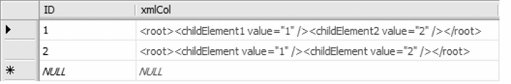The xml data type methods are described in the following subsections.

#### 7.3.1. query( )

The xml data type query( ) method queries an xml data type instance and returns an untyped xml data type instance. The query( ) syntax is:

`     query(XQuery  ) `

where:

XQuery

An XQuery expression that queries for XML nodes in an xml data type instance

The following example uses the query( ) method to extract the childElement2 element from the xml data type instance in the column xmlCol for ID = 1:

`     SELECT xmlCol.query('/root/childElement2')     FROM xmldtmTable     WHERE ID = 1 `

The result set is shown in Figure 7-6.

##### Figure 7-6. Result set for query( ) method example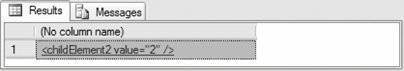#### 7.3.2. value( )

The xml data type value( ) method performs a query against an xml data type instance and returns a scalar value of SQL data type. The value( ) method syntax is:

`     value(XQuery, SQLType) `

where:

XQuery

An XQuery expression that retrieves data from the xml data type instance. An error is returned if the expression does not return at least one value.

SQLType

A string literal of the SQL data type to be returned. SQLType cannot be an xml, CLR UDT, image, text, ntext, or sql_variant data type.

The value( ) method uses the T-SQL CONVERT function implicitly to convert the result of the XQuery expression to the SQL data type.

The following example uses the value( ) method to extract the attribute value from the xml data type instance in the column xmlCol for ID = 1:

`     SELECT xmlCol.value('(/root/childElement2/@value)', 'int') Value     FROM xmldtmTable     WHERE ID = 1 `

The result set is shown in Figure 7-7.

##### Figure 7-7. Result set for value( ) method example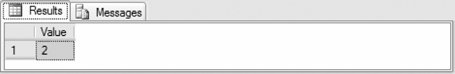The value( ) operator requires a single operand, so  is required to specify the first childElement2. The value attributes could be accessed for other childElement2 elements, if they existed, using the appropriate index.

#### 7.3.3. exist( )

The xml data type exist( ) method returns a value indicating whether an XQuery expression against an xml data type instance returns a nonempty result set. The return value is one of the following:

1

The XQuery expression returns at least one XML node.

0

The XQuery expression returns an empty result set.

NULL

The xml data type instance against which the query is executed is NULL.

The exist( ) method syntax is:

`     exist (XQuery) `

where:

XQuery

An XQuery expression

The following example uses the exist( ) method to determine whether the attribute value from the xml data type instance in the column xmlCol is a specified value for ID = 1:

`     SELECT xmlCol.exist('/root/childElement2[@value=1]')     FROM xmldtmTable     WHERE ID = 1 `

The result is shown in Figure 7-8.

##### Figure 7-8. Result for exist( ) method example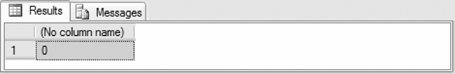The value of 0 means that the attribute value does not have the value 1. If the exist( ) method is changed to exist('/root/childElement2[@value=2]'), the result is 1.

#### 7.3.4. modify( )

The xml data type modify( ) method modifies the content of an xml data type instance. The modify( ) method syntax follows:

`     modify (XML_DML) `

where:

XML_DML

An XML Data Manipulation Language statement. The XML DML statement inserts, updates, or deletes nodes from an xml data type instance.

The modify( ) method can only be used in the SET clause of an UPDATE statement.

XML DML and the modify( ) method are discussed in more detail in the "XML Data Manipulation Language" section later in this chapter.

#### 7.3.5. nodes( )

The xml data type nodes( ) method shreds an xml data type instance into relational data by identifying nodes that will be mapped to a new row. The nodes( ) syntax is:

`     nodes (XQuery) as Table(Column) `

where:

XQuery

An XQuery expression that constructs nodes that are subsequently exposed as a result set

Table

The table name for the result set

Column

The column name for the result set

The following example uses the nodes( ) method to return the value attributes for each child element childElement as an int:

`     SELECT T.C.value('@value', 'int') AS Value     FROM xmldtmTable     CROSS APPLY xmlCol.nodes('/root/childElement') AS T(C)     WHERE ID = 2 `

The result set is shown in Figure 7-9.

##### Figure 7-9. Result set for nodes( ) method example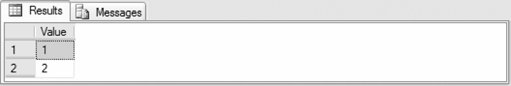The CROSS APPLY operator lets you invoke the nodes( ) method for each row returned by the query.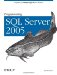Programming SQL Server 2005
ISBN: 0596004796
EAN: 2147483647
Year: 2007
Pages: 147
Authors: Bill Hamilton